Introduction to Exponents

 Problem Set 1 Problem Set 2 Problem Set 3Select a problem set using the buttons above, then use your mouse or tab key to select a question. Fill in the blank with the correct answer for each problem. When you have answered all of the questions, ask Charlie how you did.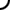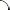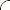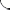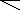Complete each multiplication operation.   2 • 2 • 2 • 2 • 2 = 2 3x • 3x • 3x • 3x = ( )4   Complete each multiplication operation.   53 • 54 = 5 x2 • x3 = 5   Complete each sum.   y3 + y3 + y3 + y3 = 4 • y b2 + b2 + b2 = • b2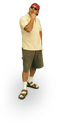Complete each multiplication operation.   (-4)(-4)(-4) = (-4) (x + 3)(x + 3) = ( )2 Complete each multiplication operation.   7 • 75 = 7 z6 • z3 = 9 Complete each sum.   a6 + a6 + a6 = 3 • a x + x + x + x = • xComplete each multiplication operation.   (m + n - 1)(m + n - 1) = (m + n - 1) (7y)(7y)(7y)(7y)(7y) = ( )5 Complete each multiplication operation.   70 • 74 = 7 (x - 1) • (x - 1) • (x - 1) = ( )3 Complete each sum.   5 + 5 + 5 = • 5 y2 + y2 + y2 = 3 • y©  2009  LarryPerezWeb Design:  Patrick Quigley